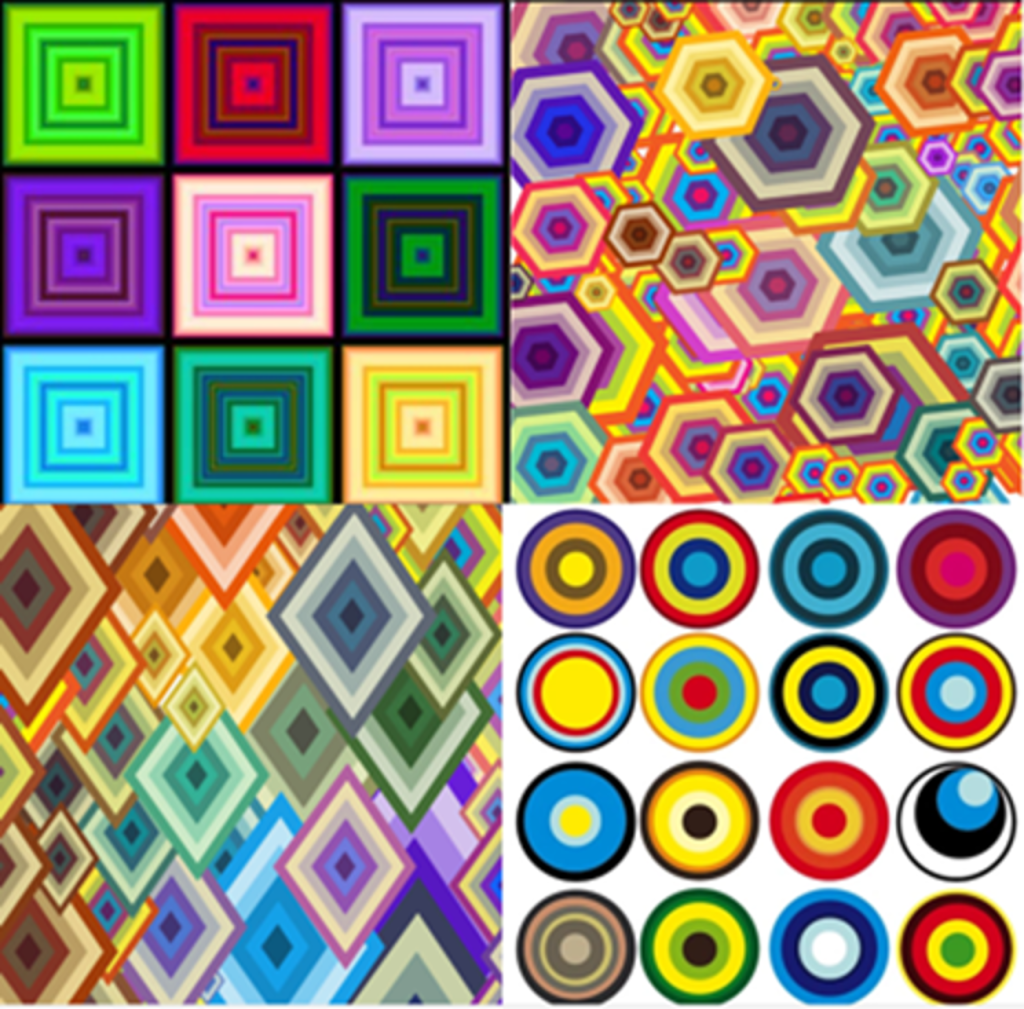# Problem of the Week## Perimeter to Area Ratio

What happens to the ratio of the area of these shapes compared to their perimeter, as they increase in size?

2D-shapes are described as similar if they have the same shape but are not the same size.

All regular 2D shapes are similar to each other eg. all squares are similar, all regular hexagons are similar. Also, all circles are also similar.

All rhombuses are not similar, but the rhombuses in this picture are all similar because the angle sizes within each of the rhombuses is the same.

When a shape is enlarged (made bigger) or reduced (made smaller), all the lengths within the shape are made larger (or smaller) by the same ratio. This ratio is called the scale factor.

e.g. If the length of each side of a square is doubled (multiplied by a scale factor of 2), the perimeter of that square is doubled.  If the length of each side of a square is tripled (multiplied by a scale factor of 3), the perimeter of that square is tripled etc.

The area of a square is length x width.

If length and width are doubled (so perimeter is multiplied by 2), the area is multiplied by 4. This is because the area becomes (2 x length) x (2 x width) = 4 x (length x width).

If length and width are tripled (so perimeter is multiplied by 3, the area is multiplied by 9. This is because the area becomes (3 x length) x (3 x width) = 9 x (length x width).

So as a shape get larger, the perimeter is multiplied by the scale factor (eg. 3) while the area is multiplied by the square of the scale factor (eg. 3 x 3 = 9).

The area is therefore going up at a higher rate than the perimeter, so the ratio of the area to the perimeter increases as the size of the shape increases.